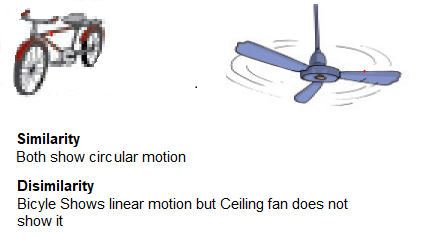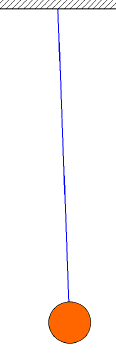# NCERT Solutions for Class 6 Science Chapter 10: Motion and Measurement of distances

In this page we have NCERT Solutions for Class 6 Science Chapter 10: Motion and Measurement of distances. Hope you like them and do not forget to like , social share and comment at the end of the page.
Question 1
Give two examples each, of modes of transport used on land, water and air.
 On Land Bicycle, Car, Bus, train On Water Ship, Motor boat, Ferry On Air Aero plane, Glider, Helicopter

Question 2
Fill in the blanks:
(i) One metre is _____ cm.
(ii) Five kilometre is ____ m. (1 Km = 1000m)
(iii) Motion of a child on a swing is ____.
(iv) Motion of the needle of a sewing machine is ___.
(v) Motion of wheel of a bicycle is___.
1. 100
2. 5000
3. Periodic Motion
4. Periodic Motion
5. Circular Motion

Question 3
Why can a pace or a footstep not be used as a standard unit of length?
A footstep not be used as a standard unit of length because the size of pace and footstep vary from person to person. This will lead to confusion while measuring the lengths by different persons. So We should use standard units like International System of Units (SI Units).

Question 4
Arrange the following lengths in their increasing magnitude:
1 metre, 1 centimetre, 1 kilometre,1 millimetre
1 millimetre < 1 centimetre < 1 metre < 1 kilometre

Question 5
The height of a person is 1.65 m. Express it into cm and mm.
As 1m = 100cm and 1cm = 10mm
So, we can say that
$1.65 m = 1.65 \times 100cm = 165cm$
Also, $1.65m = 165cm = 165 \times 10mm = 1650 mm$

Question 6
The distance between Radha’ s home and her school is 3250 m. Express this distance into km.
Since $1 \; m = \frac {1}{1000} \; km$
So, $3250 \; m = \frac {3250}{1000} = 3.250 \; km$

Question 7
While measuring the length of a knitting needle, the reading of the scale at one end is 3.0 cm and at the other end is 33.1 cm. What is the length of the needle?
Length of the needle will be given by
$= 33.1 \; cm - 3.0 \; cm = 30.1 \; cm$

Question 8
Write the similarities and differences between the motion of a bicycle and a ceiling fan that has been switched on.
 Similarity Both ceiling fan and bicycle exhibit circular motion. Difference Bicycle shows rectilinear motion while ceiling fan does not.Question 9
Why could you not use an elastic measuring tape to measure distance? What would be some of the problems you would meet in telling someone about a distance you measured with an elastic tape?

Since the tape is stretchable, its measurements will be inaccurate. Therefore, we cannot use it as measuring tape. While measuring a distance, we need to tell someone how much tape has been stretched which is difficult to measure. It leads to incorrect measurements.

Question 10
Give two examples of periodic motion.
The two examples are PendulumChild on a swing### Practice Question

Question 1 What is $\frac {1}{2} + \frac {3}{4}$ ?
A)$\frac {5}{4}$
B)$\frac {1}{4}$
C)$1$
D)$\frac {4}{5}$
Question 2 Pinhole camera produces an ?
A)An erect and small image
B)an Inverted and small image
C)An inverted and enlarged image
D)None of the above